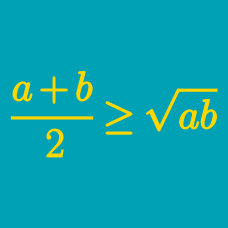Algebra

# AM-GM

Suppose the average of $5$ positive numbers is equal to $2.$ What is the maximum possible product of the numbers?

Suppose $xyz = 8$ with $x, y, z > 0.$ What is the minimum value of $x + y + z$?

What is the largest $n$ for which

$x^3 + y^3 + z^3 \geq nxyz$

holds for all $x, y, z > 0$?

What is the largest $n$ for which

$(x + y)(y + z)(x + z) \geq nxyz$

holds for all positive $x, y, z$?

Suppose $2x + 3y + z = 18.$ Find the maximum value of $xyz$ for $x, y, z > 0.$

×